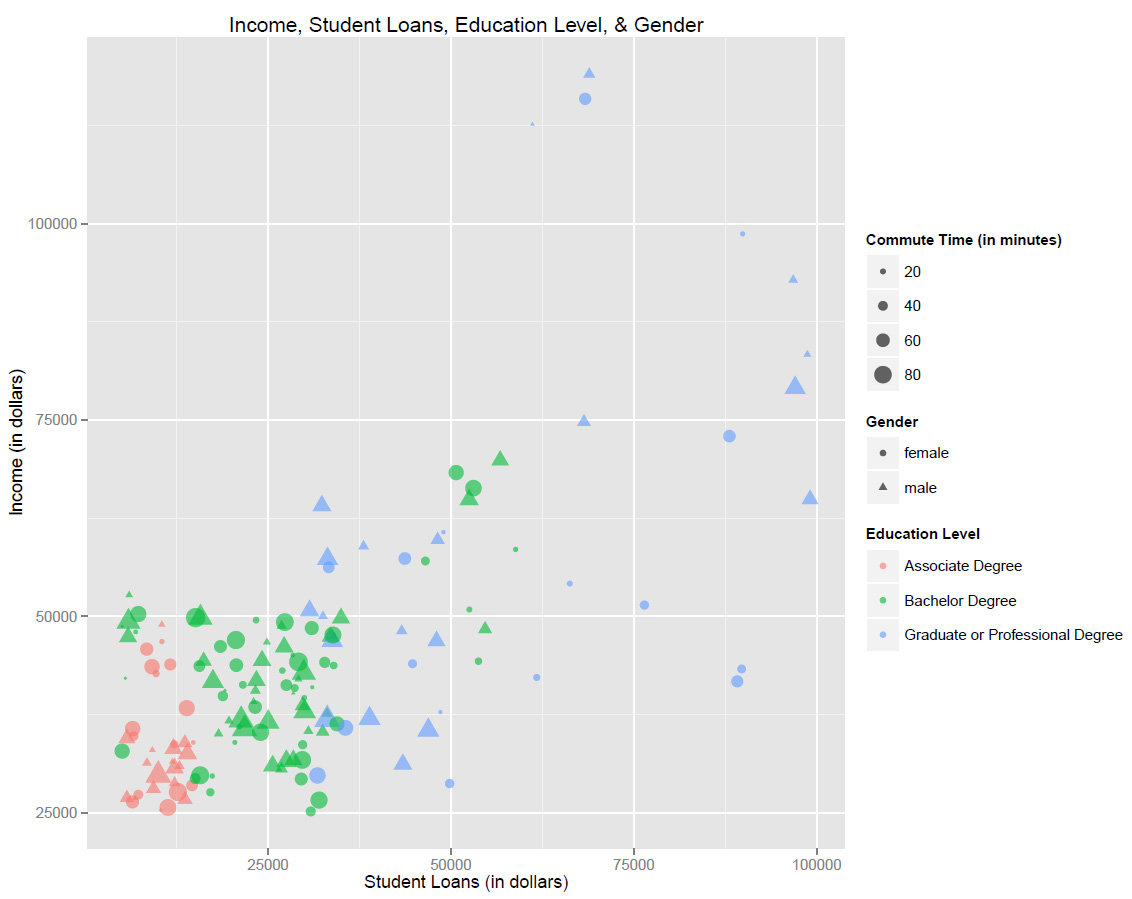# Visualizing Data with R and ggplot2

Lately, I have been spending more time playing around with R. As an R beginner and someone interested in data visualization, one of my favorite packages so far is ggplot2. This package vastly simplifies the process of plotting data and the results are rather aesthetically pleasing. One of the really powerful features of ggplot2 is the way in which it makes visually encoding multiple dimensions of a dataset much easier.

In this brief tutorial, I will plot some data generated using Excel. The data (available here) represent 150 individuals and contains information on their gender, income, time spent commuting to work, student loans, and education level. I fabricated the data so that patterns will emerge in the resulting visualization that mimic what you might expect to see in the real world, but the data are totally fake.

The following presupposes some basic familiarity with R. If you are brand new, you may want to start with a basic R tutorial – there are dozens freely available on the internet.

The first step is to install ggplot2:

install.packages("ggplot2")

require(ggplot2)

Before reading the data into R, it is helpful to set the working directory to the location where the data are saved. Here, I set the working directory to a folder on my Desktop called data:

setwd("C:/Users/dapolley/Desktop/data")

Now, read in the CSV file containing the sample data using the read.csv function:

This creates a dataframe called data that has 200 observations and six variables. The next step is to create a basic scatter plot comparing individuals’ student loans to their income:

ggplot(data, aes(x = student_loans, y = income)) +

geom_point()

The first line specifies the dataset that we are using, followed by the aesthetics of the plot. In this case, we want to see student loan values on the x-axis and income on the y-axis. The next line adds a layer to the plot specifying the geometric shape to be used. Since we want to create a scatter plot we will use geom_point(). There are a variety of different geometric shapes available in ggplot2, such as geom_bar() for bar charts.

Let’s visually encode another variable by coloring the points based on education level. We add color to the aesthetics of the plot:

ggplot(data, aes(x = student_loans, y = income, color = factor(education_level))) +

geom_point()

For discrete variables, such as education level or gender, it is important to include factor. Now, change the shape of the points to indicate gender:

ggplot(data, aes(x = student_loans, y = income, color = factor(education_level), shape = factor(gender))) +

geom_point()

We can size the points based on time spent commuting. There is no need to include factor, since time spent commuting is a continuous variable:

ggplot(data, aes(x = student_loans, y = income, color = factor(education_level), shape = factor(gender), size = commute_time)) +

geom_point()

Notice that some of the points are difficult to see due to overlapping. Add opacity to the points to clearly see all points. By including the alpha function in the geom layer, we avoid an unnecessary legend on the final visualization:

ggplot(data, aes(x = student_loans, y = income, color = factor(education_level), shape = factor(gender), size = commute_time)) +

geom_point(alpha = 0.6)

Finally, add a title and change the labels for the axes and legends:

ggplot(data, aes(x = student_loans, y = income, color = factor(education_level), shape = factor(gender), size = commute_time)) +

geom_point(alpha = 0.6) +

labs (x = "Student Loans (in dollars)", y = "Income (in dollars)", color =

"Education Level", shape = "Gender", size = "Commute Time (in minutes)") +

ggtitle("Income, Student Loans, Education Level, & Gender")

The resulting chart looks something like this:I won't offer any analysis of the resulting visualization since the data are fake, but the data and R script with comments are available https://github.iu.edu/dapolley/blog-post-ggplot2">here for those who would like to recreate this visualization themselves. This tutorial just barely sratches the surface of what is possible with this package. Gggplot2 allows for the creation of dozens of geometric objects, transforms data with a variety of statistical analysis, and much more. It is even possible to visualize geographic data using this package. I strongly recommend delving further into ggplot2.

TP

Blog Categories:
Digital Scholarship Blogs
Updated Mar 15, 2016 by Social Sciences & Digital Publishing Librarian